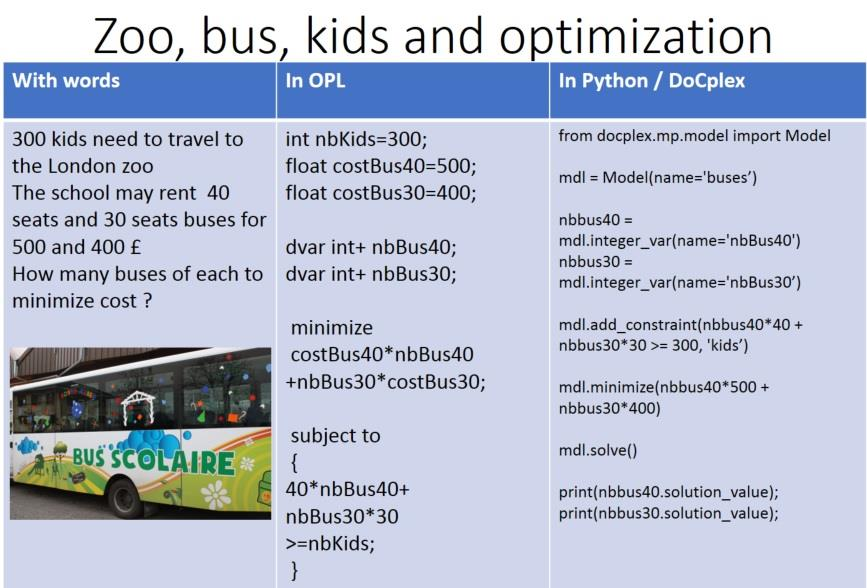# Decision Optimization

View Only

## How to add a collection index to a decision variable？#### Junye LiaoTue June 21, 2022 09:59 AM#### ALEX FLEISCHERWed June 22, 2022 01:46 AM• #### 1.  How to add a collection index to a decision variable？

Posted Tue June 21, 2022 09:59 AM
Hi,
I need a help. I need to solve my problem by using CPLEX.I would like to know how the 'y' decision variable is represented in OPL.I wrote the following, but the decision variable will report an error.
{int} C[i in 1..N] = ...;

int z[i in 1..N] = ...;
int N = ...;
dvar boolean y[i in 1..N][c in C[i]];

I know how to solve the C[I] in the constraint, but the decision variable 'y' is causing me difficulties.
subject to{
forall(i in 1..N)
sum(c in C[i])y[i][c] == z[i];
}
I would like to ask you what to do if you encounter a set in the index of a decision variable?
I am the first to post here, if I have not clear expression and expression is wrong, I hope you cross-examine and criticism. Thank you.------------------------------
Junye Liao
------------------------------

• #### 2.  RE: How to add a collection index to a decision variable？

Posted Wed June 22, 2022 01:46 AM
Hi

in a dvar array sub dimensions cannot change according to the previous ones so you can use a union:

``````int N=3;

{int} C[i in 1..N] = {i,i+1};
int z[i in 1..N] = i;
{int} bigC=union(i in 1..N) C[i];
dvar boolean y[i in 1..N][c in bigC];

subject to{
forall(i in 1..N)
sum(c in C[i])y[i][c] <= z[i];
}​``````

For more options see array variable indexer size - 3 ways : union , tuple set, decision expression in https://www.linkedin.com/pulse/making-decision-optimization-simple-alex-fleischer/------------------------------
[Alex] [Fleischer]
[Data and AI Technical Sales]
[IBM]
------------------------------

• #### 3.  RE: How to add a collection index to a decision variable？

Posted Wed June 22, 2022 09:07 PM
I'm also trying to do it using a tuple set. Thank you very much for your help!

------------------------------
Junye Liao
------------------------------